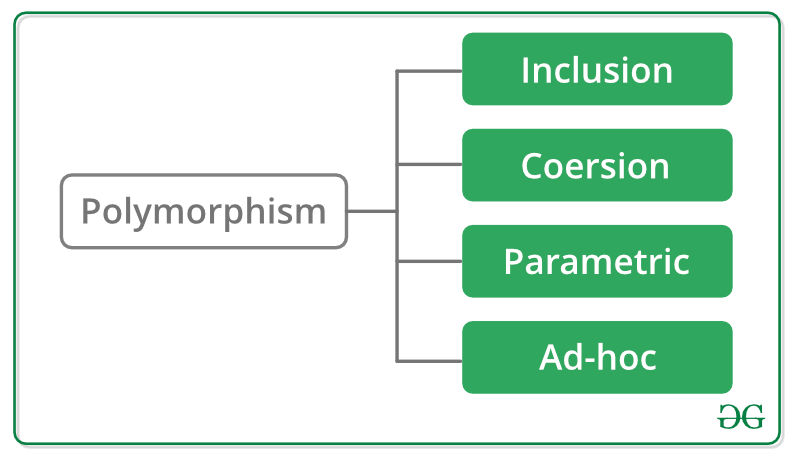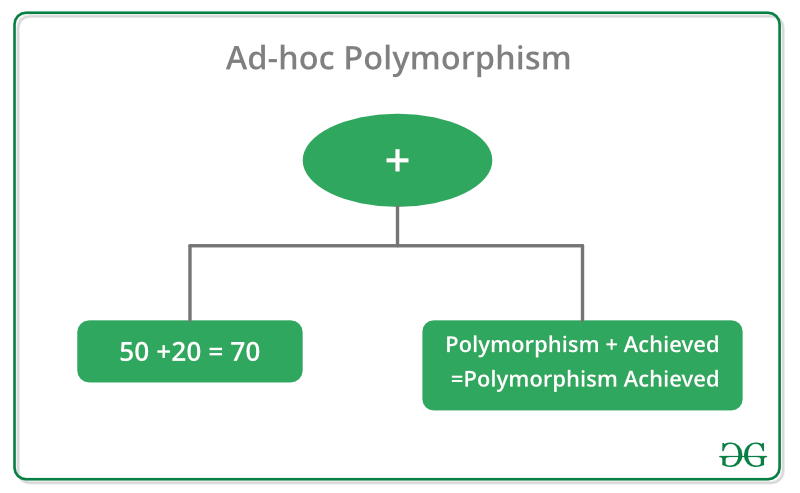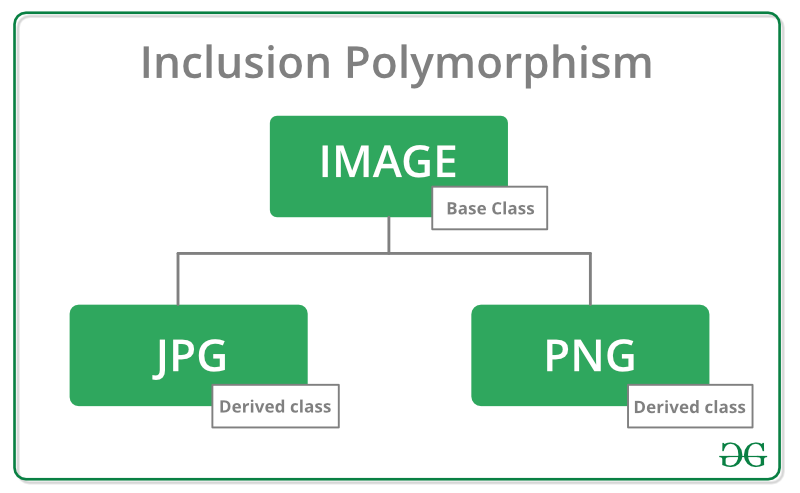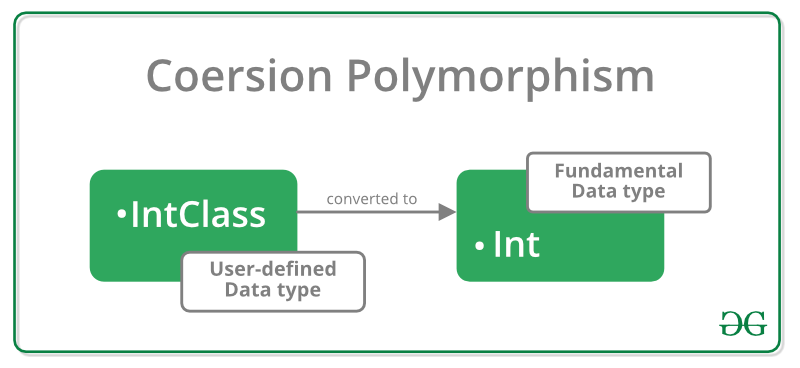# Ad-hoc, Inclusion, Parametric & Coercion Polymorphisms

• Difficulty Level : Expert
• Last Updated : 15 Jul, 2019

When we talk about Polymorphism in C++, we come to hear the following four types:Discussing these in details:

Ad-hoc Polymorphism allows functions having same name to act differently for different types. For example:
The + operator adds two integers and concatenates two strings.Above example could be better illustrated by invoking the function “sum()” in under-mentioned code:

 `#include ` `using` `namespace` `std; ` ` `  `int` `sum(``int` `x, ``int` `y) ` `{ ` `    ``int` `c = x + y; ` `    ``return` `c; ` `} ` ` `  `string sum(``const` `char``* x, ``const` `char``* y) ` `{ ` `    ``string summation(x); ` `    ``summation += y; ` `    ``return` `summation; ` `} ` ` `  `int` `main() ` `{ ` `    ``cout << sum(50, 20) ` `         ``<< ``" :- Integer addition Output\n"``; ` `    ``cout << sum(``"Polymorphism"``, ``" achieved"``) ` `         ``<< ``" :- String Concatenation Output\n"``; ` `} `

Output:

```70 :- Integer addition Output
Polymorphism achieved :- String Concatenation Output
```

Hence, by calling two different functions(which differ in the type of arguments) having the same names, to execute multiple operations, we have successfully achieved Ad-hoc Polymorphism.

2. Inclusion Polymorphism, also called as Subtyping

Inclusion Polymorphism is the ability to use derived classes through base class pointers and references. It is also known as Run-time polymorphism because the address of the function is not located by the Compiler at compile-time, rather, the right pointer from the virtual table is dereferenced to invoke the function at run-time.
The concept of Virtual Function, also known as Dynamic Linkage, is employed to achieve Inclusion Polymorphism. The usage of Virtual Function allows the selection of that function which is to be invoked based on the kind of object for which it is called.
For example:
To implement such a Polymorphism technique, let us take different files under consideration such as .jpg, .gif, .png files. All these files fall under the category of Image Files.So, they can be represented as Classes Derived from Image Base Class and overriding the display() pure virtual function. Above example could be better understood by the following illustration:

 `#include ` `using` `namespace` `std; ` ` `  `class` `Image { ` ` `  `public``: ` `    ``Image() ` `    ``{ ` `    ``} ` ` `  `    ``virtual` `void` `display() = 0; ` `}; ` `class` `Jpg : ``public` `Image { ` `public``: ` `    ``Jpg() ` `    ``{ ` `    ``} ` ` `  `    ``void` `display() ` `    ``{ ` `        ``cout << ``"JPG Image File"` `<< endl; ` `    ``} ` `}; ` ` `  `class` `Png : ``public` `Image { ` `public``: ` `    ``Png() ` `    ``{ ` `    ``} ` ` `  `    ``void` `display() ` `    ``{ ` `        ``cout << ``"PNG Image File"` `<< endl; ` `    ``} ` `}; ` ` `  `// Main function ` `int` `main() ` `{ ` `    ``Image* img; ` `    ``Jpg jg; ` `    ``Png pg; ` ` `  `    ``// stores the address of Jpg ` `    ``img = &jg; ` ` `  `    ``// invoking display() func of Jpg ` `    ``img->display(); ` ` `  `    ``// stores the address of Png ` `    ``img = &pg; ` ` `  `    ``// invoking display() func of Png ` `    ``img->display(); ` ` `  `    ``return` `0; ` `} `

Output:

```JPG Image File
PNG Image File
```

Hence, in the above code, we have two different Classes with a function having the same name and not differing by Parameters, but with different implementations.

3. Coersion Polymorphism, also called as Casting

Coersion Polymorphism occurs when an object or primitive is cast into some other type. It could be either Implicit or Explicit.

Implicit casting happens as a responsibility of Compiler itself.
For example:
float f=100
(integer implicitly gets promoted to float)

Explicit casting makes use of some type-casting expressions such as const_cast, dynamic_cast, etc.
For example:
When a class defines conversion operator for some type, say “int”, then, it could be employed anywhere in the program where integer type data is expected.
Illustration Below could make it more easier to understand:This above illustration could be more clarified with help of code below:

 `#include ` `using` `namespace` `std; ` ` `  `class` `IntClass { ` `    ``int` `num; ` ` `  `public``: ` `    ``IntClass(``int` `a) ` `        ``: num(a) ` `    ``{ ` `    ``} ` ` `  `    ``operator ``int``() ``const` `    ``{ ` `        ``return` `num; ` `    ``} ``// conversion from User-defined type to Basic type ` `}; ` ` `  `void` `show(``int` `x) ` `{ ` `    ``cout << x << endl; ` `} ` ` `  `int` `main() ` `{ ` `    ``IntClass i = 100; ` `    ``show(746); ``// outputs 746 ` `    ``show(i); ``// outputs 100 ` `} `

Output:

```746
100
```

The IntClass reference is used in place of integer type argument, and hence, the concept of Casting is well understood.

4. Parametric Polymorphism, also called as Early Binding

Parametric Polymorphism opens a way to use the same piece of code for different types. It is implemented by the use of Templates.
For example:
To develop an understanding of this sort of polymorphism, let us execute a program for finding greater of two Integers or two Strings,

 `#include ` `#include ` `using` `namespace` `std; ` ` `  `template` `<``class` `temp> ` `temp greater(temp a, temp b) ` `{ ` `    ``if` `(a > b) ` `        ``return` `a; ` `    ``else` `        ``return` `b; ` `} ` ` `  `int` `main() ` `{ ` `    ``cout << ::greater(55, 11) << endl; ` ` `  `    ``string str1(``"Early"``), str2(``"Binding"``); ` `    ``cout << ::greater(str1, str2) << endl; ` `} `

Output:

```55
Early
```

Using Templates, the same function can be parameterized with different types of data, but this needs to be decided at compile-time itself, and hence, this polymorphism is named so.
If we wish to achieve such polymorphism for pointers, it turns into Ad-hoc Polymorphism.

My Personal Notes arrow_drop_up
Recommended Articles
Page :